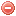# Description

The Angström index is a very simple fire index developed in Sweden and designed in order to be calculated mentally. It only requires relative humidity and temperature as input variables (Chandler et al. 1983).

It has been used in some parts of Scandinavia for indicating expected fire starts on a given day (Chandler et al. 1983).

A drawback of the Angström index is the inaccurate reflection of the relationship between relative humidity, temperature and fuel moisture (Chandler et al. 1983).

# Formula

The Anström index $$I$$
is calculated as follows (Chandler et al. 1983, Langholz & Schmidtmayer 1993):

$I=\bigg(\dfrac{H_{13}}{20}\bigg)+\bigg(\dfrac{27-T_{13}}{10}\bigg)$

where $$H_{13}$$ relative air humidity [%] and $$T_{13}$$ air temperature [°C] at 13:00.

The Angström index is supposed to be calculated on a daily basis. No particular values or conditions are required when starting the index calculation.

# Index interpretation

The Angström index is interpreted as follows:

Index values Interpretation
$$I$$ > 4.0 Fire occurrence unlikely
4.0 < $$I$$ < 2.5 Fire conditions unfavorable
2.5 < $$I$$ < 2.0 Fire conditions favorable
$$I$$ < 2.0 Fire occurrence very likely

Chandler et al. (1983)
Langholz & Schmidtmayer (1993)

### Bibliography SearchNo results!

No results were found. Are you sure you searched for a tag?

### Symbols

 Variable Description Unit $$T$$ air temperature °C $$T_{dew}$$ dew point temperature °C $$H$$ air humidity % $$P$$ rainfall mm $$U$$ windspeed m/s $$w$$ days since last rain (or rain above threshold) d $$rr$$ days with consecutive rain d $$\Delta t$$ time increment d $$\Delta{e}$$ vapor pressure deficit kPa $$e_s$$ saturation vapor pressure kPa $$e_a$$ actual vapor pressure kPa $$p_{atm}$$ atmospheric pressure kPa $$PET$$ potential evapotranspiration mm/d $$r$$ soil water reserve mm $$r_s$$ surface water reserve mm $$EMC$$ equilibrium moisture content % $$DF$$ drought factor - $$N$$ daylight hours hr $$D$$ weighted 24-hr average moisture condition hr $$\omega$$ sunset hour angle rad $$\delta$$ solar declination rad $$\varphi$$ latitude rad $$Cc$$ cloud cover Okta $$J$$ day of the year (1..365/366) - $$I$$ heat index - $$R_n$$ net radiation MJ⋅m-2⋅d-1 $$R_a$$ daily extraterrestrial radiation MJ⋅m-2⋅d-1 $$R_s$$ solar radiation MJ⋅m-2⋅d-1 $$R_{so}$$ clear-sky solar radiation MJ⋅m-2⋅d-1 $$R_{ns}$$ net shortwave radiation MJ⋅m-2⋅d-1 $$R_{nl}$$ net longwave radiation MJ⋅m-2⋅d-1 $$\lambda$$ latent heat of vaporization MJ/kg $$z$$ elevation m a.s.l. $$d_r$$ inverse relative distance Earth-Sun - $$\alpha$$ albedo or canopy reflection coefficient - $$\Delta$$ slope of the saturation vapor pressure curve kPa/°C $$Cc$$ cloud cover eights $$ROS$$ rate of spread m/h $$RSF$$ rate of spread factor - $$WF$$ wind factor - $$WRF$$ water reserve factor - $$FH$$ false relative humidity - $$FAF$$ fuel availability factor - $$PC$$ phenological coefficient -

 Suffix Description $$-$$ mean / daily value $$_{max}$$ maximum value $$_{min}$$ minimum value $$_{12}$$ value at 12:00 $$_{13}$$ value at 13:00 $$_{15}$$ value at 15:00 $$_{m}$$ montly value $$_{y}$$ yearly value $$_{f/a}$$ value at fuel-atmosphere interface $$_{dur}$$ duration $$_{soil}$$ value at soil level

 Constant Description $$e$$ Euler's number $$\gamma$$ psychrometric constant $$G_{SC}$$ solar constant $$\sigma$$ Stefan-Bolzmann constant